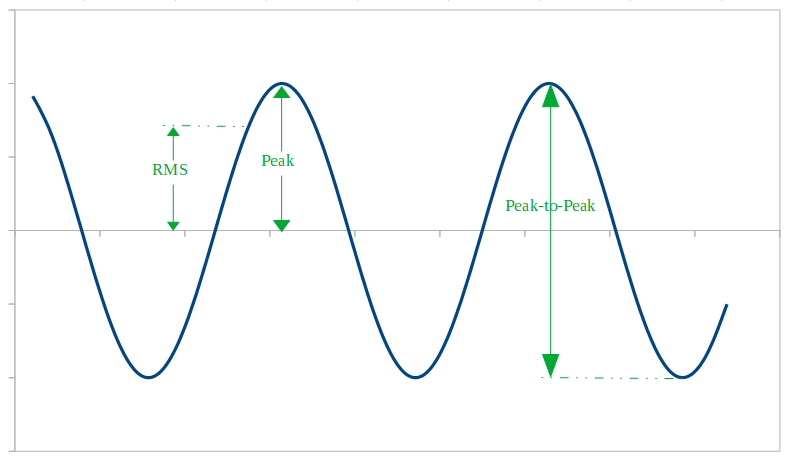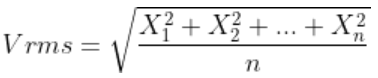# RMS Calculator. RMS, peak to peak and maximum value of a sine wave

## Calculator

 KnownParameter Parameter Value RMS Value Peak to Peak Value Maximum Value (peak value)

## Theory### Sine waves

A sine wave or sinusoid is a periodic wave in which the amplitude is proportional to the sine of its displacement angle. The electrical voltage in our households is a sine wave, and we can find sine waves in many other fields, like sound propagation, radio transmission, etc.

### RMS Value (effective value)

The average value of a sine wave (considering a complete cycle) is zero, because the area above the horizontal axis during the positive half cycle is the same as the area below the axis during the negative half cycle, so they cancel each other out. Instead of the average value, the RMS (Root-Mean-Squared) value is usually used. In the case of a set of n values, RMS is:So, it is always a positive value, even if some Xi values are negative.

In the case of AC electrical circuits, RMS value is very useful and usually voltage and current are indicated in RMS value. The AC Ohm's law is V= I · Z, where V and I are the rms values of the voltage and current and Z is the impedance

### Vp - Maximum instantaneous value (or Peak Value)

Maximum instantaneous value measured from the zero level.

### Vpp - Peak to peak value

Difference between positive and negative peaks.